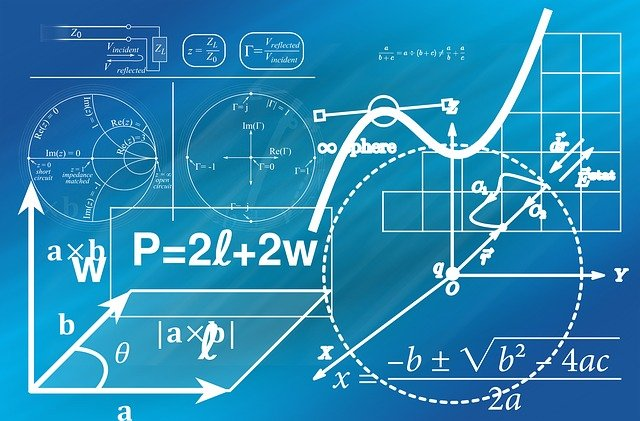image credit: pixabay

# Can we find the impossible in mathematics?

Impossibility is serious business for mathematicians. Confronted with a challenge, mathematicians will work to prove that something is possible, or find some conclusive evidence that proves it isn’t. Finding the impossible in maths is just as instructive as proving possibility, and some of the most significant results in maths emerged as a way of proving impossibility. Here’s a potted look through the history of mathematical impossibility.

You start by assuming a certain premise is true, and then proceed to derive a series of conclusions based on its truth

How do we actually prove something is impossible? One of the key tricks in the mathematician’s toolbox is the proof by contradiction – essentially, you start by assuming a certain premise is true, and then proceed to derive a series of conclusions based on its truth. Eventually, your proof will lead to a result in which two mutually contradictory things would be true, such as a number being both even and odd. The existence of such a contradiction implies that your original premise is therefore impossible. One of the most famous examples of this proof is the demonstration that the square root of two is an irrational number.

When maths was emerging during antiquity, proofs of the impossible were very much frowned upon. In the fifth century BCE, Hippasus of Metapontum discovered that it was impossible to find a line segment that can be placed end-to-end which would measure both the side and the diagonal of a regular pentagon. We know nowadays that the length of a diagonal of a regular pentagon with side length 1 is irrational, but that didn’t concur with the Pythagorean credo of “all is number”. According to legend, Hippasus was either banished or drowned for his discovery.

Several famous geometric problems emerged during this period, including the question of whether it was possible to trisect an angle with just a compass and a ruler, or square the circle. But although these problems were constructed with a compass, a new form of maths was required to actually prove they were impossible. In 1837, Pierre Wantzel used the nascent field of algebra to prove that it was impossible to use the classical tools to solve these problems, and in 1882, Ferdinand von Lindemann demonstrated that л is not constructible, and thus the circle could not be squared.

Was it possible to chart a route that crossed every one of the city’s bridges once and only once, without missing any bridge?

A similar example is the well-known ‘seven bridges of Königsberg’ problem. In 1735, a Prussian mayor approached mathematician Leonard Euler with this question – was it possible to chart a route that crossed every one of the city’s bridges once and only once, without missing any bridge. Euler realised that all that mattered in this problem were the connections, and his solution was ingeniously simple. Each time we enter and leave a land mass, we must cross two bridges – thus, for a round trip to be possible, there must be an even number of bridges. Königsberg doesn’t, and so the solution is impossible. Breaking down the problem this way is the first instance of what would come to be known as graph theory.

The issue, of course, is assuming that a problem (possible or not) is impossible simply because we don’t have the maths to deal with it at the moment. This was true of the famous Fermat’s Last Theorem, which states that there are no positive integers a, b and c which satisfy the equation an + bn = cn for any integer value of n greater than 2. It was conjectured by Pierre de Fermat around 1637 and, although it was generally believed that it was a true statement, the first proof did not emerge until 1995. Attempting to categorically prove the theorem kicked life into algebraic number theory and encouraged many mathematicians to try and resolve the modularity theorem, with significant maths advances stemming from many of these efforts.

Impossibility lingers on nowadays in the form of the Millennium Problems. These seven problems are among the most difficult in maths, with no hint of a solution (or even the possibility of one) on the cards – they may prove to be impossible, or we may need some as-of-yet undeveloped maths to resolve them, but trying to find out will undoubtedly expand our knowledge anyway.